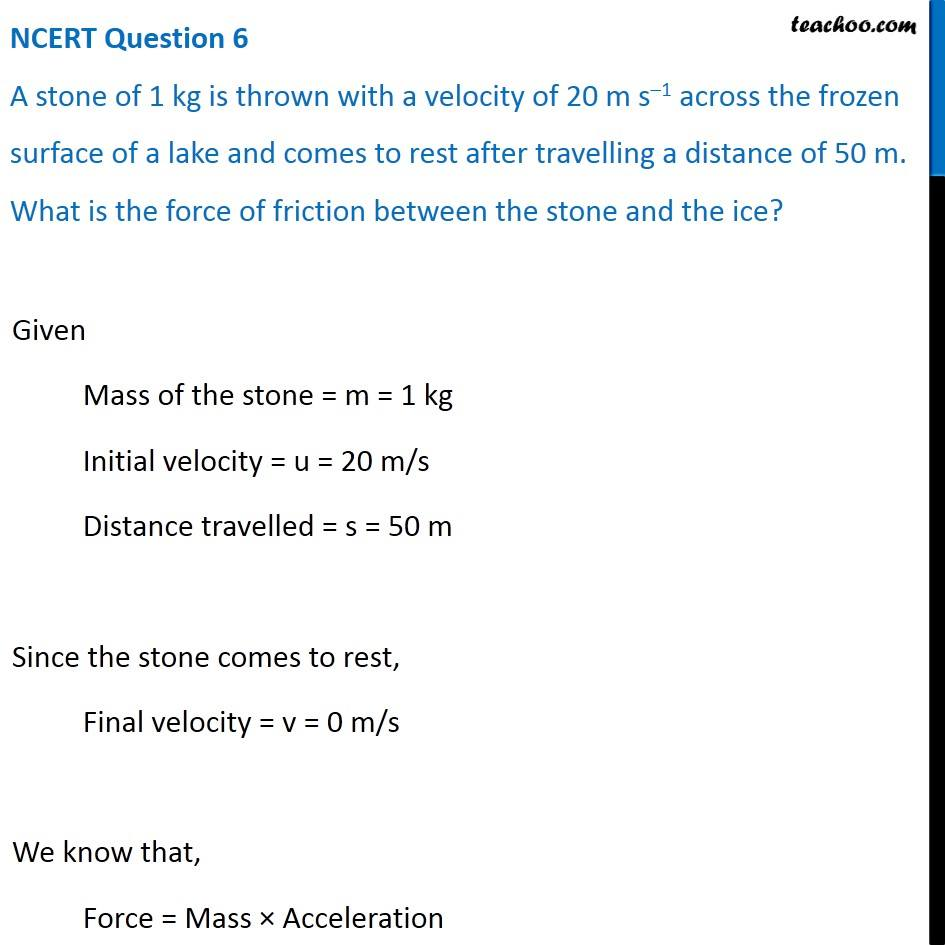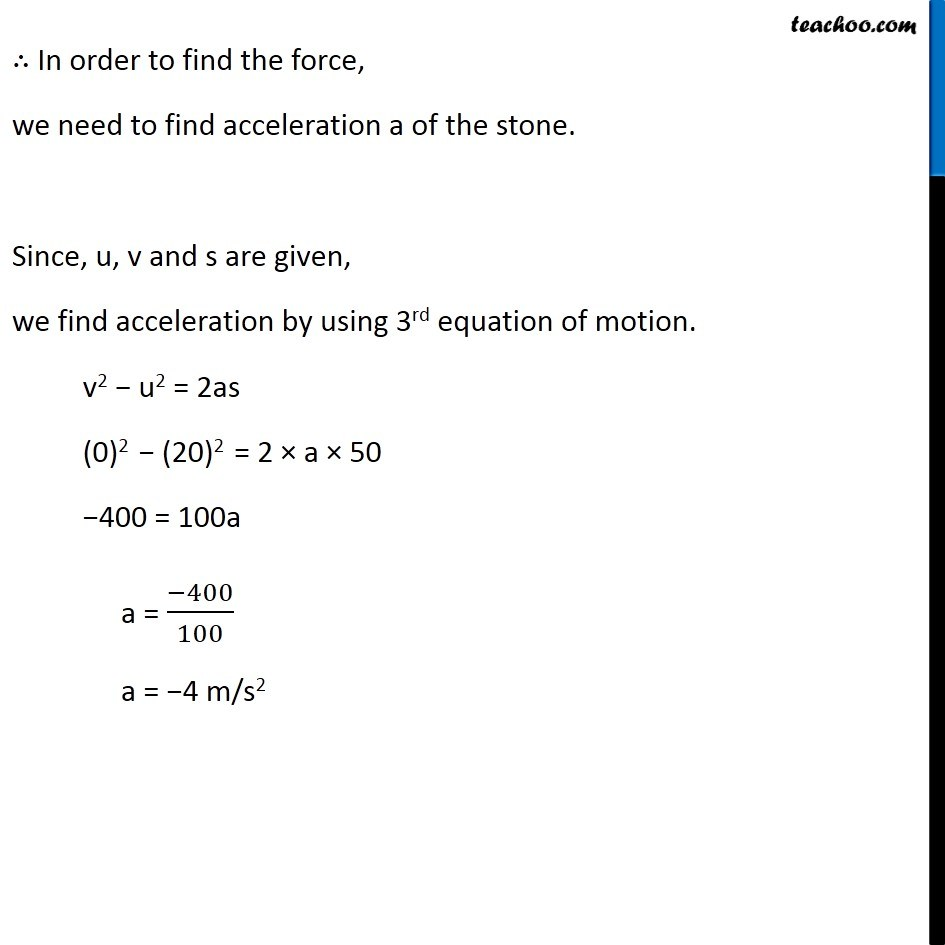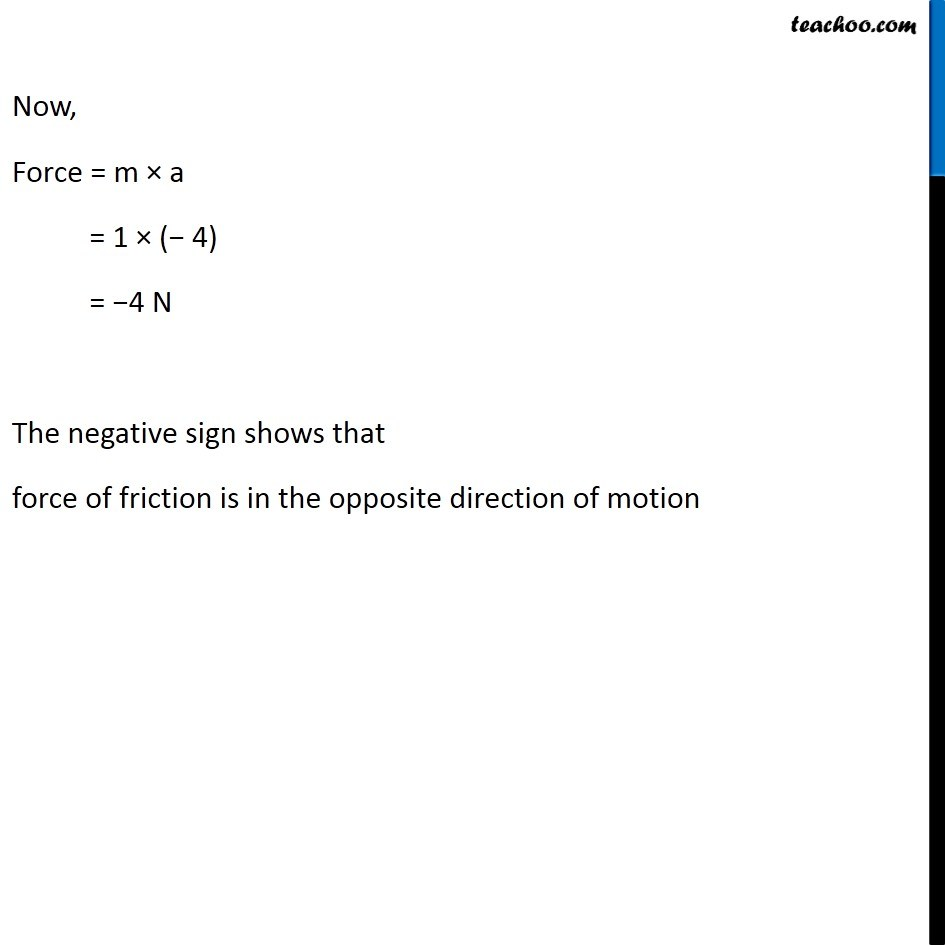NCERT Questions

Class 9
Chapter 9 Class 9 - Force and Laws Of MotionGet live Maths 1-on-1 Classs - Class 6 to 12

### Transcript

NCERT Question 6 A stone of 1 kg is thrown with a velocity of 20 m s–1 across the frozen surface of a lake and comes to rest after travelling a distance of 50 m. What is the force of friction between the stone and the ice? Given Mass of the stone = m = 1 kg Initial velocity = u = 20 m/s Distance travelled = s = 50 m Since the stone comes to rest, Final velocity = v = 0 m/s We know that, Force = Mass × Acceleration ∴ In order to find the force, we need to find acceleration a of the stone. Since, u, v and s are given, we find acceleration by using 3rd equation of motion. v2 − u2 = 2as (0)2 − (20)2 = 2 × a × 50 −400 = 100a a = (−400)/100 a = −4 m/s2 Now, Force = m × a = 1 × (− 4) = −4 N The negative sign shows that force of friction is in the opposite direction of motion